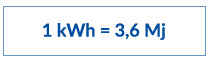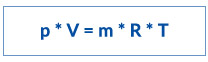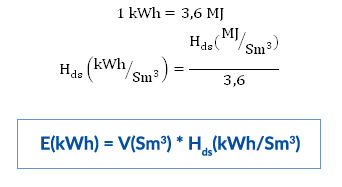# Conversion of units of measurement

1. Conversion of natural gas energy from kWh to MJ

By definition, power is the rate of doing work, i.e. consumed energy per unit time.In terms of units of measurement, the following applies:

1 W = 1 J/s
1 kW = 1000 W = 1000 J/s
1 kWh = 1000 J

If we consider the fact that 1 h = 3600 s (60 min x 60 s), i.e. 1 s = 1/3600 h, it follows that

1 kWh / 3600 = 1000 J
1 kWh = 1000 x 3600 J
1 kWh = 3600000 J

Considering that 1 MJ = 1000000 J = 106 J, it follows that2. Conversion of natural gas volume from Sm3 to kWh

Physical properties of natural gas depend on pressure, temperature and density of gas. For natural gas, the following general (ideal) gas equation applies:p - gas pressure (Pa), 1 Pa = 1 N/m2, 1 bar = 10000 Pa = 105 Pa
V- gas volume (m3)
m – gas mass (kg)
R – individual gas (or gas mixture) constant (J/kgK)
T – gas temperature (K), ϑ - gas temperature (°C), ϑ (°C) = T (K) – 273.15

Concept of “Standard cubic meter” (Sm3) represents 1 m3 of a gas at standard pressure of 101305 Pa (1.01325 bar) and temperature of 288.15 K (15 °C). For the purposes of calculating the volume of natural gas needed for accounting and determining of calorific value of gas, one uses standard conditions of gas pressure and temperature.
In its composition, natural gas is a mixture of the following gases: methane, ethane, propane, butane, nitrogen, carbon dioxide, hydrogen sulphide and other gases. The largest percentage belongs to methane (CH4), approximately 93% of mass fraction. Among other constituents, natural gas also comprises water (H2O), the chemical composition of which is unfavourable for combustion of natural gas and for its final calorific value. Consequently, two physical quantities that characterize the calorific value of natural gas with and without water vapour have been introduced, specifically:

LHV - lower heating value of natural gas (MJ/Sm3) and
HHV - higher heating value of natural gas (MJ/Sm3)

According to definition, lower heating value (LHV) represents the heat released in the process of natural gas combustion, without additional utilization of the heat of water vapour condensation. Higher heating value (HHV) represents the heat released in the process of natural gas combustion, after which the heat of condensation of water vapour from flue gases is utilized, which ultimately represents the maximum energy obtainable by gas combustion.

For the purposes of measuring the calorific value of natural gas in the process of supply of this energy source, one uses the lower heating value (LHV) as the reference value. The state-owned company Plinacro d.o.o., as the gas transmission system operator in the Republic of Croatia (TSO), is legally obligated to measure the calorific value of gas in the transmission system.  In the distribution area of Gradska plinara Zagreb d.o.o. (DSO), this is done at three locations: Zabok Pressure Reduction and Metering Station (gas imported from Slovenia coming from interconnector Rogatec), Zagreb West Pressure Reduction and Metering Station (gas coming from the Adriatic and from Slovenia) and Zagreb East Pressure Reduction and Metering Station (gas coming from gas fields in Eastern Croatia and imported gas coming from Hungary from interconnector Donji Miholjac). Natural gas from all sources is mixed in the pipes of the transmission system. By regulating the flow rate and pressure in the transmission system, the TSO regulates the quantity of natural gas coming from individual directions, and in doing so it affects the final calorific value of gas at metering points.

For the purposes of calculating the consumed volume of natural gas for a specific accounting period from Sm3 to kWh, one uses the following formulas:V (Sm3) – volume (quantity) of supplied natural gas for the relevant accounting period, determined by reading the gas meter.
LHVm (MJ/Sm3) – mean, lower heating value measured for the supplied natural gas in the relevant accounting period.
E (kWh) - energy of the supplied quantity of natural gas in the relevant accounting period.

3. Conversion of natural gas volume from Sm3 to kg

Compressed natural gas (CNG) is sold in kilos (HRK/kg). Natural gas density is measured in kg/Sm3. Based on the measurements and monthly reports on the quality and content of natural gas, which reports are delivered to the suppliers by DSO via TSO for each accounting period, one receives information about gas density, which is approximately 0.707 kg/Sm3. The formula for converting of standard cubic meter (Sm3) to kilos (kg) is the following: